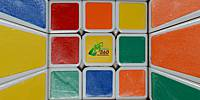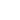Numbers ◀ Prev Next ▶

David SchaubertGerardo A. Sanchez

43,252,003,274,489,856,000

Merida, Venezuela

September 18

# Caption

43252003274489856000 Mathematics Permutations

The original (3×3×3) Rubik's Cube has eight corners and twelve edges. There are 8! (40,320) ways to arrange the corner cubes. Seven can be oriented independently, and the orientation of the eighth depends on the preceding seven, giving 37 (2,187) possibilities. There are 12!/2 (239,500,800) ways to arrange the edges, restricted from 12! because edges must be in an even permutation exactly when the corners are. (When arrangements of centres are also permitted, as described below, the rule is that the combined arrangement of corners, edges, and centres must be an even permutation.) Eleven edges can be flipped independently, with the flip of the twelfth depending on the preceding ones, giving 211 (2,048) possibilities.

8! x 3^7 x (12!/ 2) x 2^11 = 43,252,003,274,489,856,000 which is approximately 43 quintillion.

Algorithms In Rubik's cubers' parlance, a memorised sequence of moves that has a desired effect on the cube is called an algorithm. This terminology is derived from the mathematical use of algorithm, meaning a list of well-defined instructions for performing a task from a given initial state, through well-defined successive states, to a desired end-state. Each method of solving the Rubik's Cube employs its own set of algorithms, together with descriptions of what effect the algorithm has, and when it can be used to bring the cube closer to being solved.

Many algorithms are designed to transform only a small part of the cube without interfering with other parts that have already been solved, so that they can be applied repeatedly to different parts of the cube until the whole is solved. For example, there are well-known algorithms for cycling three corners without changing the rest of the puzzle, or flipping the orientation of a pair of edges while leaving the others intact.

Some algorithms do have a certain desired effect on the cube (for example, swapping two corners) but may also have the side-effect of changing other parts of the cube (such as permuting some edges). Such algorithms are often simpler than the ones without side-effects, and are employed early on in the solution when most of the puzzle has not yet been solved and the side-effects are not important. Most are long and difficult to memorise. Towards the end of the solution, the more specific (and usually more complicated) algorithms are used instead.

Relevance and application of mathematical group theory Rubik's Cube lends itself to the application of mathematical group theory, which has been helpful for deducing certain algorithms - in particular, those which have a commutator structure, namely XYX−1Y−1 (where X and Y are specific moves or move-sequences and X−1 and Y−1 are their respective inverses), or a conjugate structure, namely XYX−1, often referred to by speedcubers colloquially as a "setup move". In addition, the fact that there are well-defined subgroups within the Rubik's Cube group, enables the puzzle to be learned and mastered by moving up through various self-contained "levels of Difficulty". For example, one such "level" could involve solving cubes which have been scrambled using only 180-degree turns. These subgroups are the principle underlying the computer cubing methods by Thistlethwaite and Kociemba, which solve the cube by further reducing it to another subgroup.

# Location

Elevation: 1800Numbers ◀ Prev Next ▶